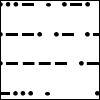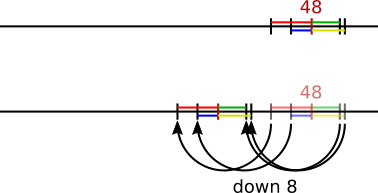You may also likeWinning Team

Nine cross country runners compete in a team competition in which there are three matches. If you were a judge how would you decide who would win?Inspector Morse

You may like to read the article on Morse code before attempting this question. Morse's letter analysis was done over 150 years ago, so might there be a better allocation of symbols today?History of Morse

This short article gives an outline of the origins of Morse code and its inventor and how the frequency of letters is reflected in the code they were given.

Shifting Average

Age 11 to 14 ShortChallenge Level

Working numerically
To find the average, the 4 numbers were added together and then divided by 4. That gave 48.

So before the total was divided by 4, it must have been 48$\times$4 = 192.

Subtracting 8 from each of the numbers will reduce the total by 4$\times$8 = 32. So the new total will be 192$-$32 = 160.

So the new average will be 160$\div$4 = 40.

Average as balancing
The average of the 4 numbers can be thought of as the point balanced between them, so that the distances above and below the average are balanced. This is shown in the diagram below - the sum of the red and blue lengths is equal to the sum of the yellow and green lengths.

So when the numbers are all reduced by 8, all of the points and the distances between them move 8 units down, as shown in the diagram. So the average will also go down by 8.So the new average is 40.

Average as sharing
Imagine that 4 people each have some money, and the average amount of money is £48. That means that if the 4 people pooled their money and then shared it out equally, they would each get £48.

If they all spend £8, that would decrease each of their shares by £8. So each person's share would be £40.

So the new average is 40.

Working algebraically
The four numbers can be written as $a,b,c$ and $d.$

Then $(a+b+c+d)\div4=48$, and we need to find $((a-8)+(b-8)+(c-8)+(d-8))\div4$.

$$\begin{split}(a-8)+(b-8)+(c-8)+(d-8)&=a+b+c+d-8-8-8-8\\ &=a+b+c+d-32\end{split}$$So $$\begin{split}((a-8)+(b-8)+(c-8)+(d-8))\div4&=(a+b+c+d-32)\div4\\ &=((a+b+c+d)\div4)-(32\div4)\end{split}$$But $(a+b+c+d)\div4=48$. So $((a+b+c+d)\div4)-(32\div4)=48-(32\div4)=48-8=40$

So the new average is $40$.

You can find more short problems, arranged by curriculum topic, in our short problems collection.